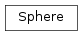# mvpa2.measures.gnbsearchlight.Sphere¶class `mvpa2.measures.gnbsearchlight.``Sphere`(radius, element_sizes=None, distance_func=None)

N-Dimensional hypersphere.

Use this if you want to obtain all the neighbors within a given radius from a point in a space with arbitrary number of dimensions assuming that the space is discrete.

No validation of producing coordinates within any extent is done.

Examples

Create a Sphere of diameter 1 and obtain all coordinates within range for the coordinate (1,1,1).

```>>> s = Sphere(1)
>>> s((2, 1))
[(1, 1), (2, 0), (2, 1), (2, 2), (3, 1)]
>>> s((1, ))
[(0,), (1,), (2,)]
```

If elements in discrete space have different sizes across dimensions, it might be preferable to specify element_sizes parameter.

```>>> s = Sphere(2, element_sizes=(1.5, 2.5))
>>> s((2, 1))
[(1, 1), (2, 1), (3, 1)]
```
```>>> s = Sphere(1, element_sizes=(1.5, 0.4))
>>> s((2, 1))
[(2, -1), (2, 0), (2, 1), (2, 2), (2, 3)]
```

Attributes

Methods

 `__call__`(coordinate) Get all coordinates within diameter `train`(dataset)

Initialize the Sphere

Parameters: radius : float Radius of the ‘sphere’. If no `element_sizes` provided – radius would be effectively in number of voxels (if operating on MRI data). element_sizes : None or iterable of floats Sizes of elements in each dimension. If None, it is equivalent to 1s in all dimensions. distance_func : None or lambda Distance function to use (choose one from `mvpa2.clfs.distance`). If None, cartesian_distance to be used.

Attributes

Methods

 `__call__`(coordinate) Get all coordinates within diameter `train`(dataset)
`distance_func`
`element_sizes`
`radius`
`train`(dataset)